# [T02] The rules of probability

For reference, here is a list of the rules of probability:
• Special addition rule (for mutually exclusive events):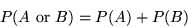• Subtraction rule: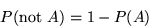• Multiplication rule: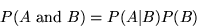• Special multiplication rule (for independent events):
It is important to bear in mind the circumstances in which the special addition rule and the special multiplication rule can be used. Most mistakes in probabilistic reasoning occur because someone assumes that events are independent when they are not (or vice versa), or because someone assumes that events are mutually exclusive when they are not (or vice versa).

Here is the original problem which led Pascal and Fermat to develop probability theory:

#### The gambler's problem

Suppose you roll a single die four times; what is the probability of rolling at least one 6? The gambler reasoned that since the chance of a 6 in each roll is 1/6, the chance of a 6 in 4 rolls is 4 x 1/6 = 2/3. Now suppose you roll a pair of dice 24 times; what is the probability of rolling at least one double 6? The gambler reasoned that since the chance of a double 6 in one roll is 1/36, the chance of a double 6 in 24 rolls is 24 x 1/36 = 2/3. In other words, the gambler expected to win each bet 2/3 of the time. His problem was that he seemed to lose more often with the second bet than the first. He was at a loss to explain this, so he asked his friend Pascal for an answer.

What are the mistakes in the gambler's reasoning? What are the true probabilities of winning each bet?

## §1. Examples and fallacies

A fallacy is a mistake in reasoning. The following examples each contain some reasoning about probabilities, some of which is correct and some of which is mistaken. See if you can spot any mistake, and then click "correct" if you think the reasoning is o.k. and "fallacy" if you think it is wrong.

• Fred is playing roulette in a Macau casino. The roulette wheel has 36 numbers (ignoring the zero), of which half are red and half are black. Fred reasons as follows: In the last ten spins, all the winning numbers have been red. But on average, only half the winning numbers are red. So to even things out, there must be more black numbers than red numbers coming up. So I stand a better chance of winning if I bet on black. or ?
• The chance of the Mark Six numbers being exactly the same two days in a row is extremely small. So to maximize my chances of winning today, I should not choose yesterday's winning numbers. or ?
• Suppose I am at the Pokfield Road bus terminus, waiting for the number 23 bus to leave for North Point. The number 23 leaves from here every 8 minutes. So the longer I wait for the bus, the higher the probability that it will leave in the next minute. or ?
• A city has a crackdown on speeding drivers, and the number of traffic fatalities falls by 12%. The local government claims that the increased enforcement has saved lives. But the crackdown was started because of a sudden increase in traffic fatalities the prior year. After an unusually high value, the number of deaths is likely to fall the following year anyway. So there is no reason to think that the crackdown caused the decrease in fatalities. or ?
• "BALTIMORE (AP) A Maryland woman this week gave birth to triplets for the second time in less than two years, defying odds of about one in 50 million, hospital officials said." The reasoning here is that since only about one birth in seven thousand is of triplets, the odds of having two sets of triplets in a row is about one in 70002, which is one in 50 million. or ?